Question

TThe Education Trust publishes data on U.S. colleges and universities. Six-year graduation rates and student-related expenditures per full-time student for 2007 were reported for the seven primarily undergraduate public universities in California with enrollments between 10,000 and 20,000. Suppose that a 90% confidence interval for the prediction of student expenditures at a university with a graduation rate of 40% was (5,304, 8,686).

Required:
a. Determine the point estimate for the prediction of student expenditures at a university with a graduation rate of 40%.
b. Calculate the margin of error.
c. Calculate the standard error of the prediction of student expenditures at a university with a graduation rate of 40%.

1.minhkhue

a) The point estimate is 6995.

b) The margin of error is of 1691.

c) The standard error is of 1000

Step-by-step explanation:

a. Determine the point estimate for the prediction of student expenditures at a university with a graduation rate of 40%.

The point estimate is the mean of the two bounds of the confidence interval. So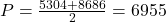The point estimate is 6995.

b. Calculate the margin of error.

The margin of error is the difference between the bounds and the point estimate. So

M = 8686 – 6995 = 6995 – 5304 = 1691

The margin of error is of 1691.

c. Calculate the standard error of the prediction of student expenditures at a university with a graduation rate of 40%.

Now I have to expand a bit into the confidence interval.

We have that to find our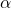level, that is the subtraction of 1 by the confidence interval divided by 2. So: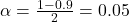Now, we have to find z in the Ztable as such z has a pvalue of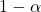.

That is z with a pvalue of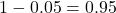, so Z = 1.645.

The margin of error is: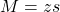In which s is the margin of error.

We have that M = 1691. So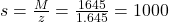The standard error is of 1000